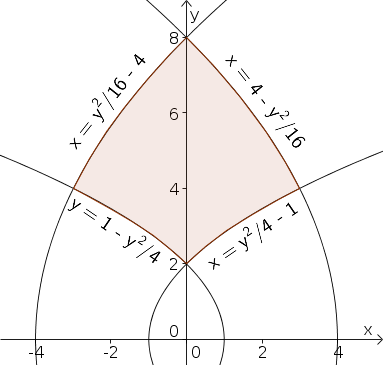# Math Insight

### Image: Example region for double integral change of variablesTo evaluate an integral over the region defined by $y > 0$, $y^2/16-4 \le x \le y^2/4-1$, $1-y^2/4 \le x \le 4-y^2/16$, one can use the change of variables $(x,y)=\cvarf(\cvarfv,\cvarsv)=(\cvarfv^2-\cvarsv^2,2\cvarfv\cvarsv)$.

Source image file: double_integral_change_variable_example_region.ggb
Source image type: Geogebra